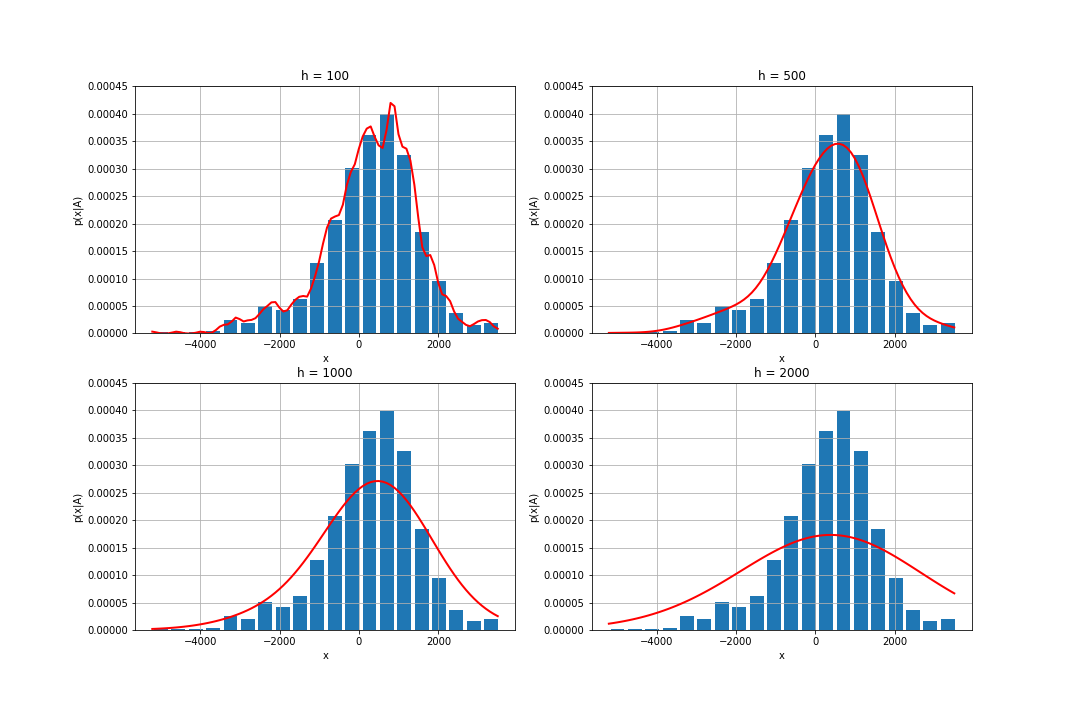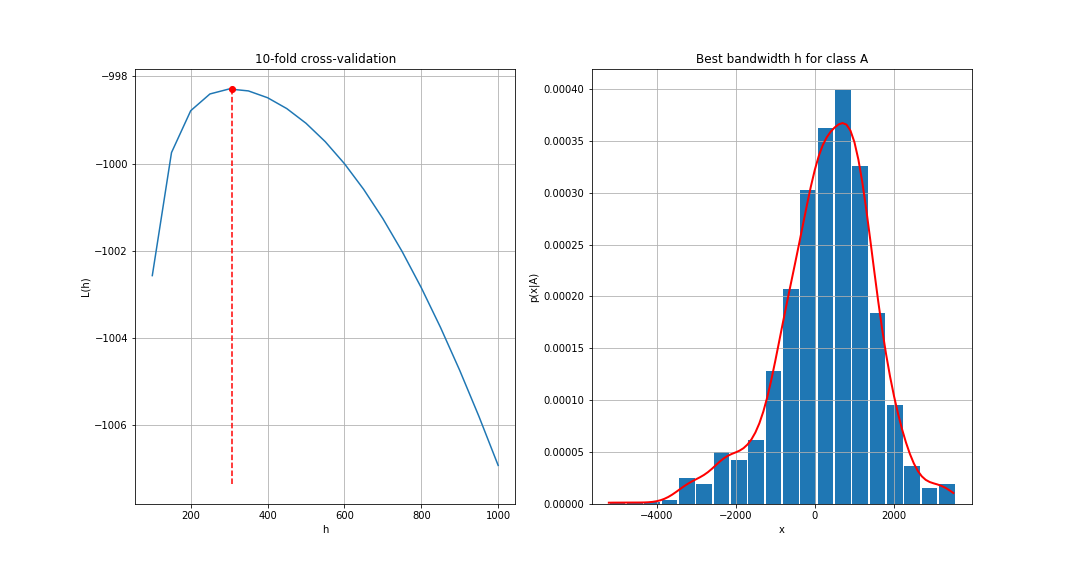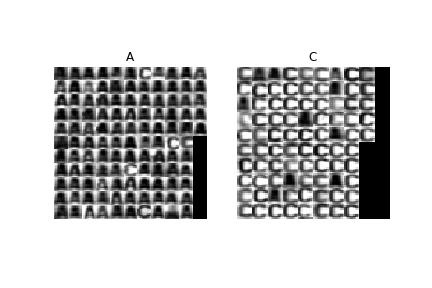# Non-parametric probability density estimation - Parzen window

As in the previous lab, we are again interested in probability density $p_{X|k}(x|k)$ estimation. Sometimes, however, one is challenged with the problem of estimating the probability density function without even knowing its parametric form (cf MLE labs). In such cases, non-parametric estimation using Parzen window method  can be applied.

The kernel density estimation $\hat{f}_h(x)$ of an unknown distribution $f(x)$ given a set $\{x_1, \ldots, x_n\}$ of independent identically distributed (i.i.d) measurements is defined as $$\hat{f}_h(x) = \frac{1}{n}\sum_{i=1}^n K_h(x - x_i)$$ where $K_h$ is the kernel (a symmetric function that integrates to one) and $h$ is the kernel size (bandwidth). Different kernel functions can be used (see ), but we will use $K_h(x) \equiv N(0, h^2)$, i.e. the normal distribution with mean 0 and standard deviation $h$.

Please, note that the kernel parameter $h$ does not make the method “parametric”. The parameter defines smoothness of the estimate, not the form of the estimated distribution. Nevertheless, the choice of $h$ still influences the quality of the estimate as you will see below. Using cross-validation  we will find such value of $h$ that ensures the estimate generalises well to previously unseen data.

Finally, using the above estimates of the probability density functions $p_{X|k}(x|k)$, we will once again build a Bayesian classifier for two letters and test it on an independent test set.

## Information for development in Python

To fulfill this assignment, you need to submit these files (all packed in one .zip file) into the upload system:

• parzen.ipynb - a script for data initialization, calling of the implemented functions and plotting of their results (for your convenience, will not be checked)
• parzen.py - containing the following implemented methods:
• my_parzen - Parzen window estimator
• compute_Lh - function for computing cross-validated log-likelihood ratio as a function of $h$
• classify_bayes_parzen - classification using Parzen window density estimates
• parzen_estimates.png - plots of estimates of $p_{X|k}(x|A)$ for varying $h$
• optimal_h_classA.png, optimal_h_classC.png - graph with optimal bandwidth $h$ for class A and C respectively
• parzen_classif.png - image with parzen classification

Use template of the assignment. When preparing a zip file for the upload system, do not include any directories, the files have to be in the zip file root.

## The tasks, part 1: Parzen window estimation

In this part we will implement the Parzen window density estimation method (see the formula above). For measurements, use the function compute_measurement_lr_cont. The data loaded in the parzen.ipynb template contain training data trn and test data tst - images and their correct labels. For both, the label 0 denotes an image of letter 'A' and label 1 an image of letter 'C'.

Do the following:

1. For each image from the training set trn compute the measurement $x$. Store the measurements for different classes separately, i.e. create two vectors xA and xC.

2. Complete the template of the function p = my_parzen(x, trn_x, h) so that for a given $x$ (possibly a vector of values) it computes the Parzen estimation of probability density $p_{X|k}(x|k)$. Here, trn_x is a vector of training measurements for one of the classes (xA or xC) and $h$ is the kernel bandwidth. As the kernel function $K_h(x)$ use the normal distribution $N(0, h^2)$.

Hint: Use scipy.stats.norm.pdf() to calculate $K_h$.

p = my_parzen(np.array([1, 2, 3]), np.array([-1, 0, 2, 1.5, 0]), 1.0)
print(p)
# -> [0.22639369 0.17268428 0.07609717]

1. Use my_parzen function to estimate the probability distribution $p_{X|k}(x|A)$. Show the normalised histogram of xA and the estimated $p_{X|k}(x|A)$ for $h \in \{100, 500, 1000, 2000\}$ in one figure (use subfigure function) and save it as parzen_estimates.png.
• To compute the estimate of $p_{X|k}(x|A)$ use your function: $p_{X|k}(x|A)$ = my_parzen(x, xA, h)
• Iterate the variable $x$ e.g. from min(xA) to max(xA), with a step of 100.## The tasks, part 2: Finding the optimal kernel size

As we have just seen, different values of $h$ produce different estimates of $p_{X|k}(x|A)$. Obviously, for $h=100$ the estimate is influenced too much by our limited data sample and it will not generalise well to unseen examples. For $h=2000$, the estimate is clearly oversmoothed. So, what is the best value of $h$?

To assess the ability of the estimate to generalise well to unseen examples we will use 10-fold cross-validation . We will split the training set ten times (10-fold) into two sets $X^i_{\mbox{trn}}$ and $X^i_{\mbox{tst}}$, $i=1,\ldots,10$ and use the set $X^i_{\mbox{trn}}$ for distribution estimation and the set $X^i_{\mbox{tst}}$ for validation of the estimate. We will use the log-likelihood of the validation set given the bandwidth $h$, $L^i(h) = \sum_{x\in X^i_{\mbox{tst}}} log(p_{X|k}(x|A))$, averaged over ten splits $L(h)=1/10 \sum_{i=1}^{10} L^i(h)$ as a measure of the estimate quality. The value of $h$ which maximises $L(h)$ is the one which produces an estimate which generalises the best. See  for further description of the cross-validation technique.

Do the following:

1. Estimate the optimal value of the bandwidth $h$ using the 10-fold cross-validation. The procedure (shown for the class A, the class C is analogous) is as follows:
1. Split the training set xA into 10 subsets using the crossval function.

2. Complete the template function Lh = compute_Lh(itrn, itst, x, h).
The function needs to compute the log-likelihood $L^i(h)$ on the each pair of training and test sets $X^i_{\mbox{trn}}$ (itrn{i}) and $X^i_{\mbox{tst}}$ (itst{i}) using the training part for estimation of $p_{X|k}(x|A)$ and the test part for computing the log-likelihood. The output is the mean log-likelihood $L(h)$ over all 10 folds.

Hint 1: $p_{X|k}(x|A) = \mbox{my_parzen}(x, X^i_{\mbox{trn}}, h)$.
Hint 2: itrn{i} and itst{i} contain only data indexes, not data themselves!

3. Plot the log-likelihood average $L(h)$ for $h = 100, 150, \ldots, 1000$. Find the optimal value of $h$ using the scipy.optimize.fminbound function and show the density estimate of $p_{X|k}(x|A)$ with the optimal $h$. Save the graphs into optimal_h_classA.png2. Repeat the previous steps to estimate the distribution of $p_{X|k}(x|C)$ (save the figure into optimal_h_classC.png.

3. Use the estimated distributions $p_{X|k}(x|A)$ and $p_{X|k}(x|C)$ to create a Bayesian classifier with zero-one loss function:

1. Estimate the a priori probabilities $p_K(A)$ and $p_K(C)$ on the training set, as you did last week.

2. Complete the template of the function labels = classify_bayes_parzen(x_test, xA, xC, pA, pC, h_bestA, h_bestC) which takes the measurements x_test computed on set tst and finds a label for each test example.

Hint: Notice, that my_parzen returns a probability for any $x$, also for $x\in \mbox{x_tst}$. No need for approximation, interpolation or similar techniques here!## Assignment Quiz

For the following questions it is assumed you run rand('seed', 42); always before the splitting of the training set (for both A and C). Subsequent uses of the random generator (including crossval function) will be unambiguously defined.

1. Is it possible to estimate $p_{X|k}(x|k)$ outside of the range of the [min(x_trn), max(x_trn)] interval?
• a) Yes
• b) No
• c) Only if we have enough data
2. What is the optimal value of $h$ for class A and class C rounded to two decimal points?
3. What is the classification error (in percents) of the Bayesian classifier rounded to two decimal points?

Solution

The kernel function will now be a 2D normal distributions with diagonal covariance matrix cov = sigma^2*eye(2). Display the estimated distributions $p_{X|k}(x|k)$.